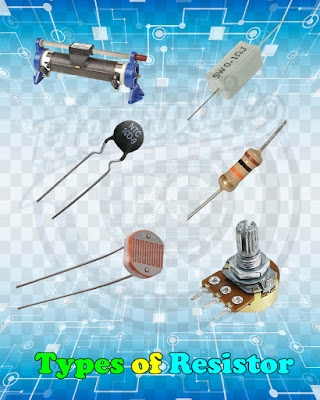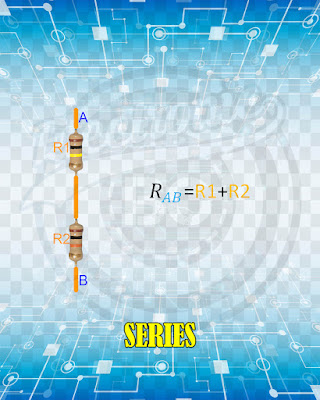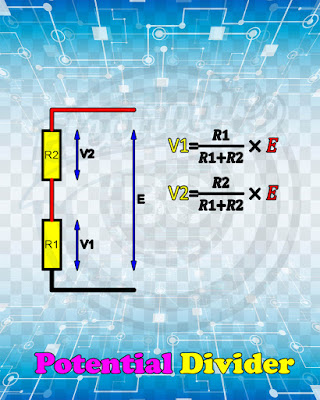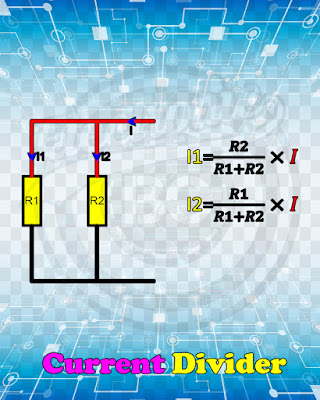Resistance is an electrical quantity that measures how the device or material reduces the electric current flow through it. The resistance is measured in units of ohms (Ω).

### Types of Resistor:Resistors are the most fundamental and commonly used of all the electronic components, to the point where they are almost taken for granted but they play a vital role within a circuit. There are many different Types of Resistor available for the electronics constructor to choose from, from very small surface mount chip resistors up to large wire wound power resistors.

### Resistors and Color Codes:The electronic color code has been used since the early 1920s. Developed by the Radio Manufacturers Association (RMA), it evolved into the color bands we see on resistors due to the fact that it was a cheap and simple way to identify small components. . Most people in the industry know of the mnemonics used to remember the color code order with one of the most popular being “Big Boys Race Our Young Girls But Violet Generally Wins”, which correspond to the colors; Black, Brown, Red, Orange, Yellow, Green, Blue, Violet, Gray, and White, respectively. Obviously, this does not account for the tolerance bands Gold, Silver, and None (no band). There are literally hundreds of different mnemonics, some of which include these missing bands. It is up to the user to pick whatever sticks in their head the best.

### Use Ohms Law:Look at the drawing above and see if it makes sense to you that:

If you increase the voltage in a circuit while the resistance is the same, you get more current.
If you increase the resistance in a circuit while the voltage stays the same, you get less current.

Ohm’s law is a way of describing the relationship between the voltage, resistance and current using math:

E = R*I

E: is the symbol for voltage.
I: is the symbol for current.
R: is the symbol for resistance.

### Resistors in Series and Parallel:

#### Connected in Series:When resistors are connected ‘ end-to-end ’ so that the same current flows through them all they are said to be cascaded or connected in series .

Resistors in series: R=R1+R2+R3+… ohm

#### Connected in Parallel:When resistors are joined ‘ side-by-side ’ so that their corresponding ends are connected together they are said to be connected in parallel .

Resistors in parallel: 1/R=1/R1+1/R2+1/R3+... siemens

### Potential and Current Divider Theory:

#### Potential Divider Theory:When resistors are connected in series the p.d. developed across each resistor will be in direct proportion to its resistance value. This is a useful fact to bear in mind, since it means it is possible to calculate the p.d.s without first having to determine the circuit current.

Potential divider: V1=(R1/R1+R2)*E volt

#### Current Divider Theory:when resistors are connected in parallel the total circuit current divides between the alternative paths available. So far we have determined the branch currents by calculating the common p.d. across a parallel branch and dividing this by the respective resistance values. However, these currents can be found directly, without the need to calculate the branch p.d.

Current divider: I1=(R2/R1+R2)*I amp